Courses

# Test: Thermodynamics Of Chemical Equilibrium(Chemical Equilibrium)

## 15 Questions MCQ Test Chemistry for JEE | Test: Thermodynamics Of Chemical Equilibrium(Chemical Equilibrium)

Description
This mock test of Test: Thermodynamics Of Chemical Equilibrium(Chemical Equilibrium) for JEE helps you for every JEE entrance exam. This contains 15 Multiple Choice Questions for JEE Test: Thermodynamics Of Chemical Equilibrium(Chemical Equilibrium) (mcq) to study with solutions a complete question bank. The solved questions answers in this Test: Thermodynamics Of Chemical Equilibrium(Chemical Equilibrium) quiz give you a good mix of easy questions and tough questions. JEE students definitely take this Test: Thermodynamics Of Chemical Equilibrium(Chemical Equilibrium) exercise for a better result in the exam. You can find other Test: Thermodynamics Of Chemical Equilibrium(Chemical Equilibrium) extra questions, long questions & short questions for JEE on EduRev as well by searching above.
QUESTION: 1

### Direction (Q. Nos. 1-9) This section contains 9 multiple choice questions. Each question has four choices (a), (b), (c) and (d), out of which ONLY ONE option is correct. Q. For the reaction in equilibrium, I. CO(g) + 1/2O2(g)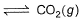II. 2CO(g) + O2 (g)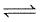2CO2 (g)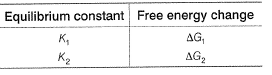Then

Solution:

CO(g) + ½ O2(g)  <====> CO2(g)
For this reaction, k1 = [CO2]/[CO][O2
2CO(g) +  O2(g)  <====> 2CO2(g)
For this reaction, k2 = [CO2]2/[CO]2[O2] = ([CO2]/[CO][O2]½)2 = (k1)2
So, k2 = k12
∆G = -RTln keqm
∆G2/∆G1 = ln k1/ln k12 = 2
∆G2 = 2∆G1

QUESTION: 2

### Equilibrium constant for the following equilibrium,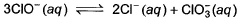is 1.0 x 1028 at a temperature at which RT = 2500 J mol-1. Gibbs free energy change (ΔG°) is

Solution:

ΔG° = -RT lnk
ΔG° = -(2500) (ln1028)
ΔG° = -161.2 kJ

QUESTION: 3

### Kp for the equilibrium  N2O4 (g)2NO2 (g)  is 0.141 at 25°C and 1 bar. This reaction is spontaneous at 25°C and partial pressures of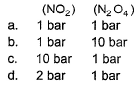Solution:
QUESTION: 4

For the following equilibrium at 373 K,
H2O(g)H2O(g)
ΔAG° is

Solution:
QUESTION: 5

For the following electrochemical cell reaction at 298 K,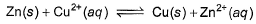E°cell = 1.10 V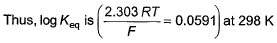Solution:

Zn(s) + Cu2+(aq) ⇌ Cu(s) + Zn2+(aq),

E= +1.10V

∴ Eo = 0.0591/n log10Keq

because at equilibrium,

Ecell = 0

(n = number of electrons exchanged = 2)

1.10 = 0.0591/2 log10Keq
2.20/0.0591 = log10Keq

Keq = antilog37.225

QUESTION: 6

Given
COCl2(g)CO(g) + Cl2 (g)
Kp = 8 x 10-9 atm
ΔS° = 30 cal K-1 at 373 K.
Temperature at which phosgene will be 0.1 % dissociated at 2 atm
(R = 2 cal mol-1K-1)is

Solution:
QUESTION: 7

For the following equilibrium at 298 K,
CO(g) + H2O (g)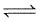CO2(g) + H2(g);
Δ f G° (in kcal mol-1) of CO = - 32.81, CO2 = - 94.26, H2O = - 54.64, H2 = 0.0 then, degree of dissociation of CO(g) is

Solution:
QUESTION: 8

At 1 atmospheric pressure, N2O4 is 50% dissociated at 300 K and 80% dissociated at 400 K as given
N2O4 (g)2NO2 (g)
Thus, enthalpy of dissociation is

Solution:
QUESTION: 9

At 800 K, ΔG° for the reaction

NiO (s) + H2(g)Ni (s) + H2O (g)

is - 9.0 kcal. Thus, ratio of pressures of water vapours and hydrogen in equilibrium with NiO and Ni at 800 K in terms of logarithm is

Solution:
QUESTION: 10

Direction (Q. Nos. 10) This sectionis based on statement I and Statement II. Select the correct answer from the code given below.

Q. Statement I

For a reaction  2NO2(g)N2O4 (g) variation of (log10 K) with (T-1) is represented as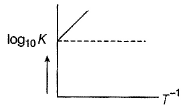Statement II

Association o f NO2 to N2O4 is an exotherm ic efiange.

Solution:
*Multiple options can be correct
QUESTION: 11

Direction (Q. No. 11) This section contains 4 multiple choice questions. Each question has four
choices (a), (b), (c) and (d), out of which ONE or  MORE THANT ONE  is correct.

Q. Variation o f equilibrium constant K with tem perature, T is given by van’t Hoff equation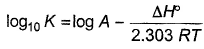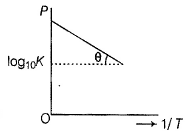Graphically it is shown as given θ = tan (0 .5) and OP = 10

Thus,

Solution:
QUESTION: 12

Direction (Q. Nos. 12-13) This section contains  a paragraph, wach describing theory, experiments, data etc. three Questions related to paragraph have been given.Each question have only one correct answer among the four given options (a),(b),(c),(d)

1 mole H2(g) and 0.2 mole CO2(g) are introduced in a vacuum flask at 450°C and 0.5 atm.

H2(g) + CO2H2O(G) + CO(g)

Analysis shows that mixture contains 10 moles per cent steam. Also equilibrium constant increases by one per cent per degree around 450°C.(log 1.1 = 0.0414)

Q. Equilibrium constant Kp is

Solution:
QUESTION: 13

1 mole H2(g) and 0.2 mole CO2(g) are introduced in a vacuum flask at 450°C and 0.5 atm.

H2(g) + CO2 (g)H2O (g) + CO (g)
Analysis shows that mixture contains 10 moles per cent steam. Also equilibrium constant increases by one per cent per degree around 450°C.(log 1.1 = 0.0414)

Q. ΔH (enthalpy change) by taking equilibrium constant at 450° C and 460° C is

Solution:
QUESTION: 14

Direction (Q. No. 14) Choice the correct combination of elements and column I and coloumn II  are given as option (a), (b), (c) and (d), out of which ONE option is correct.

The hydrogenation of pyridine (C5H5N) to piperidine (C5H11N)

C5H5N(g) + 3H2(g)C5H11N(g)

is an equilibrium process whose equilibrium constant (Kp)is given by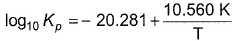Match the thermodynamics parameters in column I with their respective values in column II.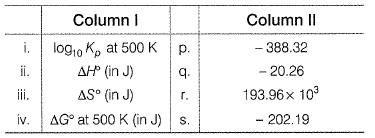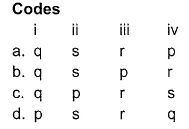Solution:
*Answer can only contain numeric values
QUESTION: 15

Direction (Q. Nos. 15) This section contains 1 questions. when worked out will result in an integer from 0 to 9 (both inclusive)

For a reversible reaction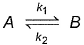Rate constant k1 (forward)  = 1015e -2000/T and k2 (backward ) = 1012e -2000/T

What is the value of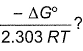Solution: